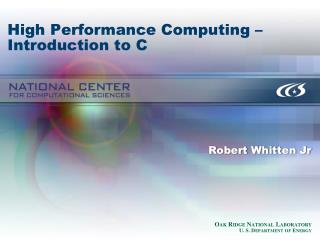DownloadDownload PresentationHigh Performance Computing – Introduction to C

# High Performance Computing – Introduction to C

Download Presentation## High Performance Computing – Introduction to C

- - - - - - - - - - - - - - - - - - - - - - - - - - - E N D - - - - - - - - - - - - - - - - - - - - - - - - - - -
##### Presentation Transcript

1. High Performance Computing – Introduction to C Robert Whitten Jr

2. Welcome! • Today’s Agenda: • Questions from last week • C language

3. From last time… • Send me that email if you haven’t already whittenrm1@ornl.gov • Apply for an account on Smoky http://www.nccs.gov/user-support/access/account-request/

4. C basics • Comments • Variables • Arrays • Selection • Loops • Functions

5. C program example – Hello World #include <stdio.h> void main(void) { printf(“Hello World\n”); }

6. Hello World + #include <stdio.h> void main(void) { int x; x=10; printf(“Hello World\n”); printf(“x=%d”,x); }

7. Hello World ++ #include <stdio.h> int main(int argc, char** argv) { printf(“Hello World, %s\n”, argv); return (0); }

8. Variables

9. Variable declaration • int I; • char c; • double dbl; • float f; • int i=0;

10. Arrays • One dimensional • int i ; • char str ; • Two dimensional • int d ;

11. Selection • if condition then something else something else. if (x < 10) { printf(“low\n”); }

12. Loops • While condition do while (x < 10) { printf(“low\n”); x++; } • Do until condition do { printf(“low\n”); x++; } while (x<10);

13. Loops • For expression do for (i=0; i<10; i++) { printf(“low\n”); }

14. Functions • Recurring pieces of code <return> func (parameters) { body }

15. Matrices: What They Are • Multidimensional grids of numbers • Properties • Can be rectangular • Can be 1D, 2D,3D, 4D, … • m x m, m x n

16. Matrices and Modeling • Matrix models related set of measures: e.g., • Counts • Costs • Physical data • Measures characterize a set of related, measurable entities (e.g., people) • Columns model properties of these entities

17. Matrices and Modeling (example) • Matrix counts social activities • Rows represent set of friends • Lynn, Mel, Noel, Pat • Columns represent activity types • Col. 1: movies • Col. 2: dinners • Col. 3: car trips • Col. 4: gifts

18. Matrices and Modeling (example) • Matrix models costof social activities (10’s of \$s) • Rows represent set of friends • Lynn, Mel, Noel, Pat • Columns represent activity types • Col. 1: movies • Col. 2: dinners • Col. 3: car trips • Col. 4: gifts

19. Matrices and Modeling (example) • Matrix models value of financial instruments (1,000s of \$s) • Rows represent institution’s depositors • Columns represent instrument type (1,000’s of \$s) • Col. 1: stocks • Col. 2: bonds • Col. 3: commodities • Col. 4: cash

20. Matrices and Modeling (example) • Matrix models counts of registered voters • Rows represent locales of interest • Column represent classes of registrations • Col. 1: Republicans • Col. 2: Democrats • Col. 3: Independents • Col. 4: Unregistered

21. Matrices and Modeling (example) • Matrix models counts of birds • Rows represent species • Columns represent counting stations

22. Matrices and Modeling (example) • Matrix models ocean temperatures (degrees Celsius) • Rows represent buoys • Columns represent points in time • Col. 1: 18 hours ago • Col. 2: 12 hours ago • Col. 3: 6 hours ago • Col. 4: now

23. Matrices and Modeling • Can flip-flop row, column semantics for these examples, e.g., • Columns represent buoys • Rows represent points in time

24. Matrices and Modeling (example) • If movies cost \$10, dinners cost \$20, car trips cost \$30, and gifts cost \$40, how much did Lynn, Mel, Noel, and Pat spend for the indicated time frame?